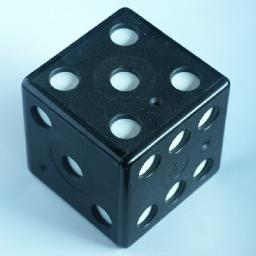# Rubik's DiceRubik's Dice consists of a hollow cube with holes where the pips of a die would be (plus a few smaller holes). Inside are 7 square white metal plates which have red dots painted on them. These plates can be manipulated so that they stick to the insides of the cube, which is helped by the magnets built into the sides. The aim is to do this in such a way that none of the holes show a red spot, just white ones.

It is patented by Jozsef Bognar and Ernő Rubik (WO 90/10481 filed on 9 March 1990, granted 10 September 1990, and earlier Hungarian patent HU 1190/89 granted on 10 March 1989).

## The number of positions:

There are 7 plates of which 6 are used to cover the sides of the die. Each plate has two sides and can be placed in 4 orientations. This gives a total of 7!·86 = 1,321,205,760 positions with 6 filled sides of which only one shows no red dots.

## Solution:

Below is a table showing the plates. Each plate has a small number printed in the corner which can be used to identify it. This is shown on the left of the table. The plates are shown in white (.) and red (X) and grey (O). The grey parts show which parts of the plates are visible in the solved position. This solution is unique. This is easy to see if the sides are solved in descending order, from 6 to 1, when there is only one remaining possibility for each side. Note how side 1 of each plate used shows the same pattern as side 2 of the plate used to solve the next lower number.

Number Side 1Side 2 Die number solved
78 . X .     O o O 6
X . X O . O
. . . O X O

65 . . .   O X O 5
X X X X O X
. X . O o O

34 X X .   O X O 4
X . . o X X
. . . O X O

58 X . .   o . O 3
. X . . O X
. . . O X X

12 . . X   O . o 2
. . . . X .
X . X X . O

14 X . X   X . o 1
. X . . O .
. . . X . X

47 . . .   . . . None
. X . . X .
X . X X . X